# Adding Two Vectors in General

Go back to  'Vectors'

Now, suppose that $$\overrightarrow a$$ and $$\overrightarrow b$$ are any two arbitrary vectors: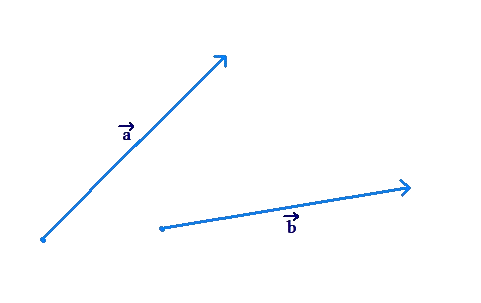How do we find the net effect of $$\overrightarrow a$$ and $$\overrightarrow b$$, or $$\overrightarrow a + \overrightarrow b$$, in this general scenario?

To answer that question, we first understand another property of vectors. A vector will remain the same no matter where it is translated, as long as its magnitude and direction are preserved. In the following figure, the leftmost arrow represents vector $$\overrightarrow a$$, while the other arrows are all translated copies of this arrow (translation means no change in either magnitude or direction):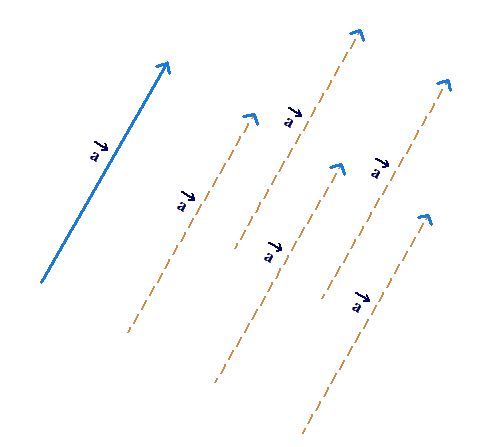The other arrows will then also represent vector $$\overrightarrow a$$. This is because they represent the same magnitude and the same direction as vector $$\overrightarrow a$$.

Thus, a vector does not change under translation. In this sense, a vector is a free entity - it can be translated around anywhere without changing it.

Now, we come back to the question of finding $$\overrightarrow a + \overrightarrow b$$ when $$\overrightarrow a$$ and $$\overrightarrow b$$ are any two arbitrary vectors. Let us translate $$\overrightarrow b$$ so that its initial point coincides with the ending point of $$\overrightarrow a$$: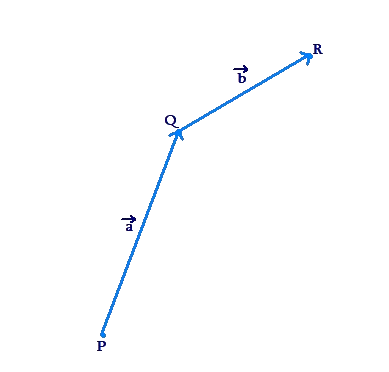Can you now visualize what the net effect of $$\overrightarrow a$$ and $$\overrightarrow b$$ will be? Think of $$\overrightarrow a$$ and $$\overrightarrow b$$ as displacement vectors for a moment. If you are at point P, the effect of $$\overrightarrow a$$ will be to take you to from P to Q. Then, the effect of $$\overrightarrow b$$ will be to take you from Q to R. Thus, the net effect of $$\overrightarrow a$$ and $$\overrightarrow b$$ will be to take you from P to R. In other words, $$\overrightarrow a + \overrightarrow b$$is the vector joining P to R: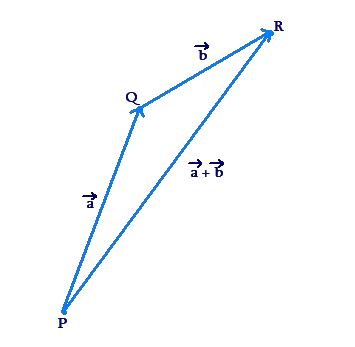This is the triangle law of vector addition. To add two vectors, make the initial point of the second vector coincide with the final point of the first, and then complete the triangle. The third side (joining the initial point of the first vector to the final point of the second vector) represents the sum of the two vectors.

Example 1: Vector $$\overrightarrow a$$ has a magnitude of 4 units and points towards the east. Vector $$\overrightarrow b$$ as a magnitude of 4 units and makes an angle of 1200 with the east direction: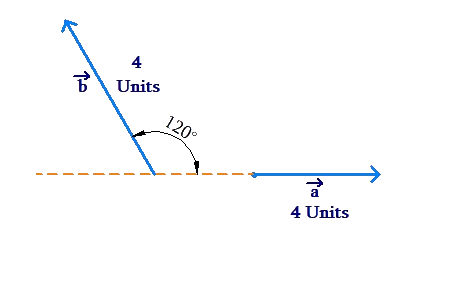Find $$\overrightarrow a + \overrightarrow b$$.

Solution: We make the starting point of $$\overrightarrow b$$ coincide with the tip of $$\overrightarrow a$$: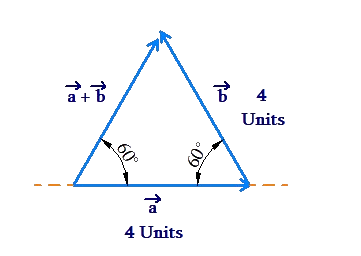Note that this triangle is equilateral. Clearly, $$\overrightarrow a + \overrightarrow b$$ is a vector of magnitude 4 units and makes an angle of 600 with the east direction.

Example 2: Vectors $$\overrightarrow a$$ and $$\overrightarrow b$$ are inclined at an angle of $$\theta$$ to each other: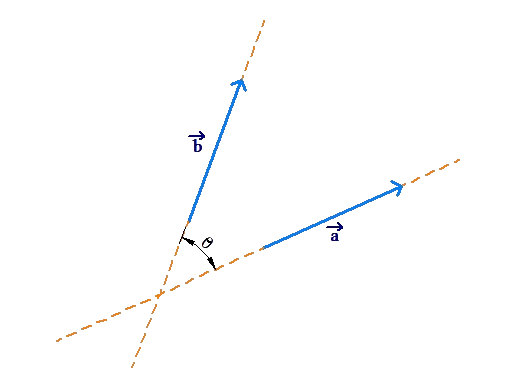Find the sum, or resultant, of these two vectors.

Solution: Make the initial point of $$\overrightarrow b$$ coincide with the tip of $$\overrightarrow a$$: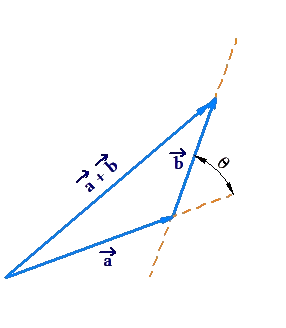Using the cosine law, the third side has a magnitude of:

$\begin{array}{l}\left| {\overrightarrow a + \overrightarrow b } \right| = \sqrt {{{\left| {\overrightarrow a } \right|}^2} + {{\left| {\overrightarrow b } \right|}^2} - 2\left| {\overrightarrow a } \right|\left| {\overrightarrow b } \right|\cos \left( {\pi - \theta } \right)} \\\,\,\,\,\,\,\,\,\,\,\,\,\, \qquad= \sqrt {{{\left| {\overrightarrow a } \right|}^2} + {{\left| {\overrightarrow b } \right|}^2} + 2\left| {\overrightarrow a } \right|\left| {\overrightarrow b } \right|\cos \theta } \end{array}$

Now, observe the following figure: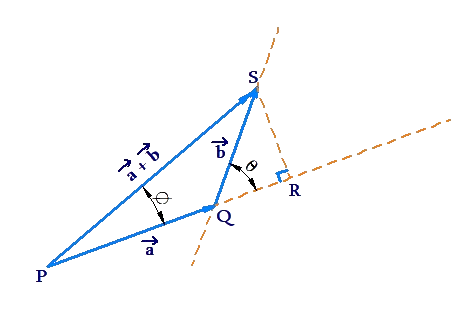Clearly, the resultant makes an angle $$\phi$$ with $$\overrightarrow a$$ given by:

$\tan \phi = \frac{{SR}}{{PR}} = \frac{{\left| {\overrightarrow b } \right|\sin \theta }}{{\left| {\overrightarrow a } \right| + \left| {\overrightarrow b } \right|\cos \theta }}$

Thus, we have determined both the magnitude as well as the direction of the resultant vector.

A slightly different variant of the triangle law is the parallelogram law of vector addition. To add two vectors $$\vec a$$ and $$\vec b$$, we first make them co-initial: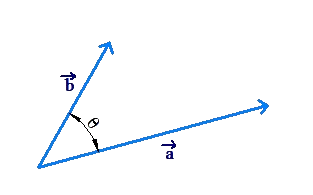Now, we complete the parallelogram with these vectors forming adjacent sides. The diagonal vector joining the initial points of the two vectors to the opposite end point of the parallelogram represents $$\vec a + \vec b$$: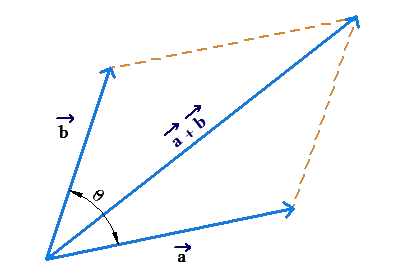Note that because a parallelogram has equal and parallel opposite sides, the parallelogram law is equivalent to the triangle law: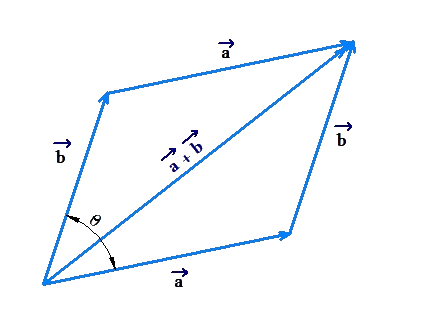Example 3: Two forces are applied on a box, as shown below: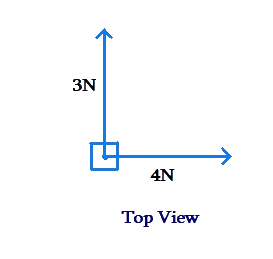Find the resultant force (the net effect of these two forces).

Solution: If we represent the 3 N force by $$\overrightarrow {{F_1}}$$ and the 4 N force by $$\overrightarrow {{F_2}}$$, we need to determine $$\overrightarrow {{F_1}} + \overrightarrow {{F_2}}$$. We make use of the parallelogram law: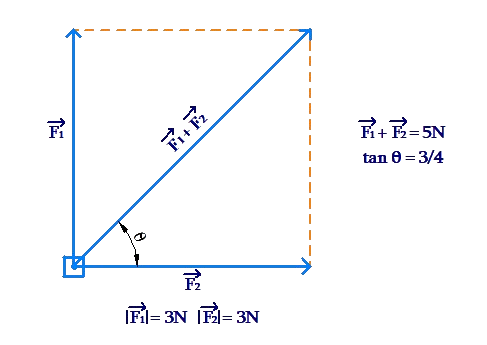Clearly, $$\overrightarrow {{F_1}} + \overrightarrow {{F_2}}$$ has a magnitude of 5 N, and is inclined at an angle of $${\tan ^{ - 1}}\frac{3}{4}$$ or about 370 to the 4 N force.

Example 4: Three equal forces (each of 5 N magnitude) are applied on a point object, inclined at an angle of 1200 to each other: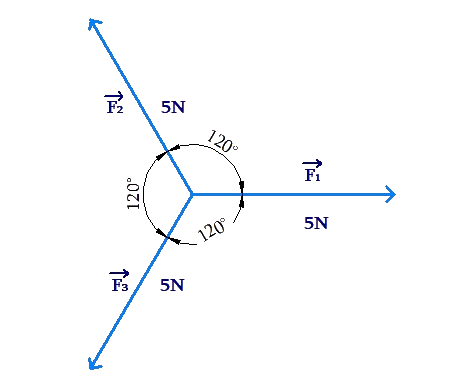Show that the net force on the object is 0 N.

Solution: We need to show that $$\overrightarrow {{F_1}} + \overrightarrow {{F_2}} + \overrightarrow {{F_3}} = \overrightarrow 0$$. Let us use the parallelogram law to calculate $$\overrightarrow {{F_{12}}}$$, the resultant of $$\overrightarrow {{F_1}}$$ and $$\overrightarrow {{F_2}}$$: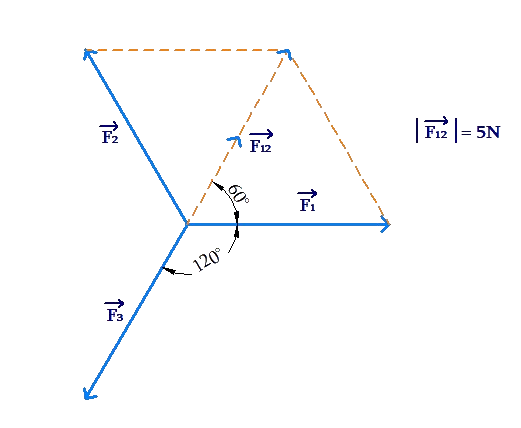Note from the figure that $$\overrightarrow {{F_{12}}}$$ has a magnitude equal to 5 N, and is directed exactly opposite to $$\overrightarrow {{F_3}}$$. Thus, the resultant of $$\overrightarrow {{F_{12}}}$$ and $$\overrightarrow {{F_3}}$$ is $$\overrightarrow 0$$. In other words:

$\overrightarrow {{F_1}} + \overrightarrow {{F_2}} + \overrightarrow {{F_3}} = \overrightarrow 0$

Example 5: (a) In a triangle ABC, show that $$\overrightarrow {AB} + \overrightarrow {BC} + \overrightarrow {CA} = \overrightarrow 0$$

(b) In a polygon $${A_1}{A_2}{A_3}...{A_n}$$, show that

$\begin{array}{l}\overrightarrow {{A_1}{A_2}} + \overrightarrow {{A_2}{A_3}} + \overrightarrow {{A_3}{A_4}} + ...\\ \qquad\;\; ... + \overrightarrow {{A_{n - 1}}{A_n}} + \overrightarrow {{A_n}{A_1}} = \overrightarrow 0 \end{array}$

Solution: (a) Consider the following figure: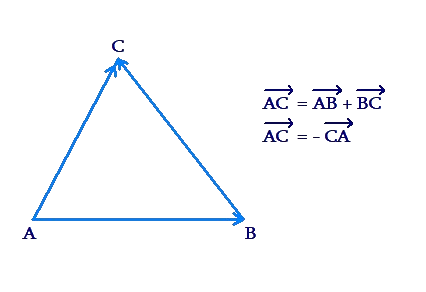Applying the triangle law, we see that

$\overrightarrow {AC} = \overrightarrow {AB} + \overrightarrow {BC}$

But also, we note that $$\overrightarrow {AC} = - \overrightarrow {CA}$$. Thus,

$\begin{array}{l} - \overrightarrow {CA} = \overrightarrow {AB} + \overrightarrow {BC} \\ \Rightarrow \,\,\,\overrightarrow {AB} + \overrightarrow {BC} + \overrightarrow {CA} = \overrightarrow 0 \end{array}$

Let us understand this result geometrically. Suppose that you are standing at the point A. A displacement of $$\,\overrightarrow {AB}$$ will take you to from the point A to the point B. Then, from B, a displacement of $$\overrightarrow {BC}$$ will take you to the point C. Finally, from C, a displacement of $$\overrightarrow {CA}$$ will bring you back to A. Thus, the net effect of $$\overrightarrow {AB}$$, $$\overrightarrow {BC}$$and $$\overrightarrow {CA}$$is a displacement of zero. Mathematically: $$\overrightarrow {AB} + \overrightarrow {BC} + \overrightarrow {CA} = \overrightarrow 0$$.

(b) We can extend the logic above to a polygon of n-sides. Starting from $${A_1}$$, a displacement of $$\overrightarrow {{A_1}{A_2}}$$ will take you to $${A_2}$$; from $${A_2}$$, a displacement of $$\overrightarrow {{A_2}{A_3}}$$ will take you to $${A_3}$$, and so on, until the final displacement $$\overrightarrow {{A_n}{A_1}}$$ takes you from $${A_n}$$ back to $${A_1}$$. Thus, the net effect of all these vectors is zero, so their sum is the zero vector.

Let us see a specific example of this result. Consider a pentagon ABCDE: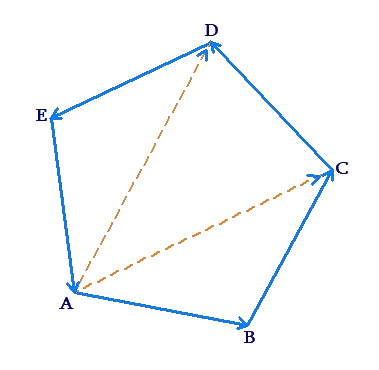Using the triangle law, note that $$\overrightarrow {AB} + \overrightarrow {BC} = \overrightarrow {AC}$$, $$\overrightarrow {AC} + \overrightarrow {CD} = \overrightarrow {AD}$$, and $$\overrightarrow {AD} + \overrightarrow {DE} = \overrightarrow {AE}$$. Thus,

$\begin{array}{l}\overrightarrow {AB} + \overrightarrow {BC} + \overrightarrow {CD} + \overrightarrow {DE} + \overrightarrow {EA} \\ = \left( {\overrightarrow {AB} + \overrightarrow {BC} } \right) + \overrightarrow {CD} + \overrightarrow {DE} + \overrightarrow {EA} \\ = \overrightarrow {AC} + \overrightarrow {CD} + \overrightarrow {DE} + \overrightarrow {EA} \\ = \left( {\overrightarrow {AC} + \overrightarrow {CD} } \right) + \overrightarrow {DE} + \overrightarrow {EA} \\ = \overrightarrow {AD} + \overrightarrow {DE} + \overrightarrow {EA} \\ = \left( {\overrightarrow {AD} + \overrightarrow {DE} } \right) + \overrightarrow {EA} \\ = \overrightarrow {AE} + \overrightarrow {EA} = \overrightarrow 0 \end{array}$

You can extrapolate the same approach to an arbitrary polygon of n sides.

Vectors
grade 10 | Questions Set 1
Vectors
Vectors